# How to Calculate and Solve for Pressure Head | Aquifer Characteristics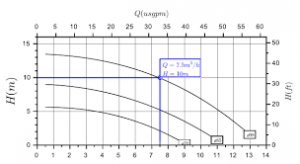The image above represents pressure head.

To compute for pressure head, three essential parameters are needed and these parameters are Pressure (P), Density (ρ) and Acceleration due to Gravity (g).

The formula for calculating pressure head:

hp = P / ρg

Where:

P = Pressure
p = Density
g = Acceleration due to Gravity

Let’s solve an example;
Find the pressure head when the pressure is 18, the density is 14 and the acceleration due to gravity is 9.

This implies that;

P = Pressure = 18
p = Density = 14
g = Acceleration due to Gravity = 9

hp = P / ρg
hp = 18 / (14)(9)
hp = 18 / 126
hp = 0.142

Therefore, the pressure head is 0.142.

Calculating the Pressure when the Pressure Head, the Density and the Acceleration due to Gravity is Given.

P = hp x pg

Where;

P = Pressure
p = Density
g = Acceleration due to Gravity

Let’s solve an example;
Find the pressure when the pressure head is 25, the density is 6 and the acceleration due to gravity is 9.

This implies that;

hp = Pressure Head = 25
p = Density = 6
g = Acceleration due to Gravity = 9

P = hp x pg
P = 25 x (6)(9)
P = 25 x 54
P = 1350

Therefore, the pressure is 1350.

Calculating the Density when the Pressure Head, the Pressure and the Acceleration due to Gravity is Given.

p = P / hp x g

Where;

p = Density
P = Pressure
g = Acceleration due to Gravity

Let’s solve an example;
Find the density when the pressure head is 4, the pressure is 48 and the acceleration due to gravity is 9.

This implies that;

hp = Pressure Head = 4
P = Pressure = 48
g = Acceleration due to Gravity = 9

p = P / hp x g
p = 48 / 4 x 9
p = 48 / 36
p = 1.33

Therefore, the density is 1.33.

Calculating the Acceleration due to Gravity when the Pressure Head, the Pressure and the Density is Given.

g = P / hp x p

Where;

g = Acceleration due to Gravity
P = Pressure
p = Density

Let’s solve an example;
Find the acceleration due to gravity when the pressure head is 10, the pressure is 40 and the density is 2.

This implies that;

hp = Pressure Head = 10
P = Pressure = 40
p = Density = 2

g = P / hp x p
g = 40 / 10 x 2
g = 40 / 20
g = 2

Therefore, the acceleration due to gravity is 2.

Nickzom Calculator – The Calculator Encyclopedia is capable of calculating the pressure head.

To get the answer and workings of the pressure head using the Nickzom Calculator – The Calculator Encyclopedia. First, you need to obtain the app.

You can get this app via any of these means:

To get access to the professional version via web, you need to register and subscribe for NGN 2,000 per annum to have utter access to all functionalities.
You can also try the demo version via https://www.nickzom.org/calculator

Apple (Paid) – https://itunes.apple.com/us/app/nickzom-calculator/id1331162702?mt=8
Once, you have obtained the calculator encyclopedia app, proceed to the Calculator Map, then click on Geology under Add-on.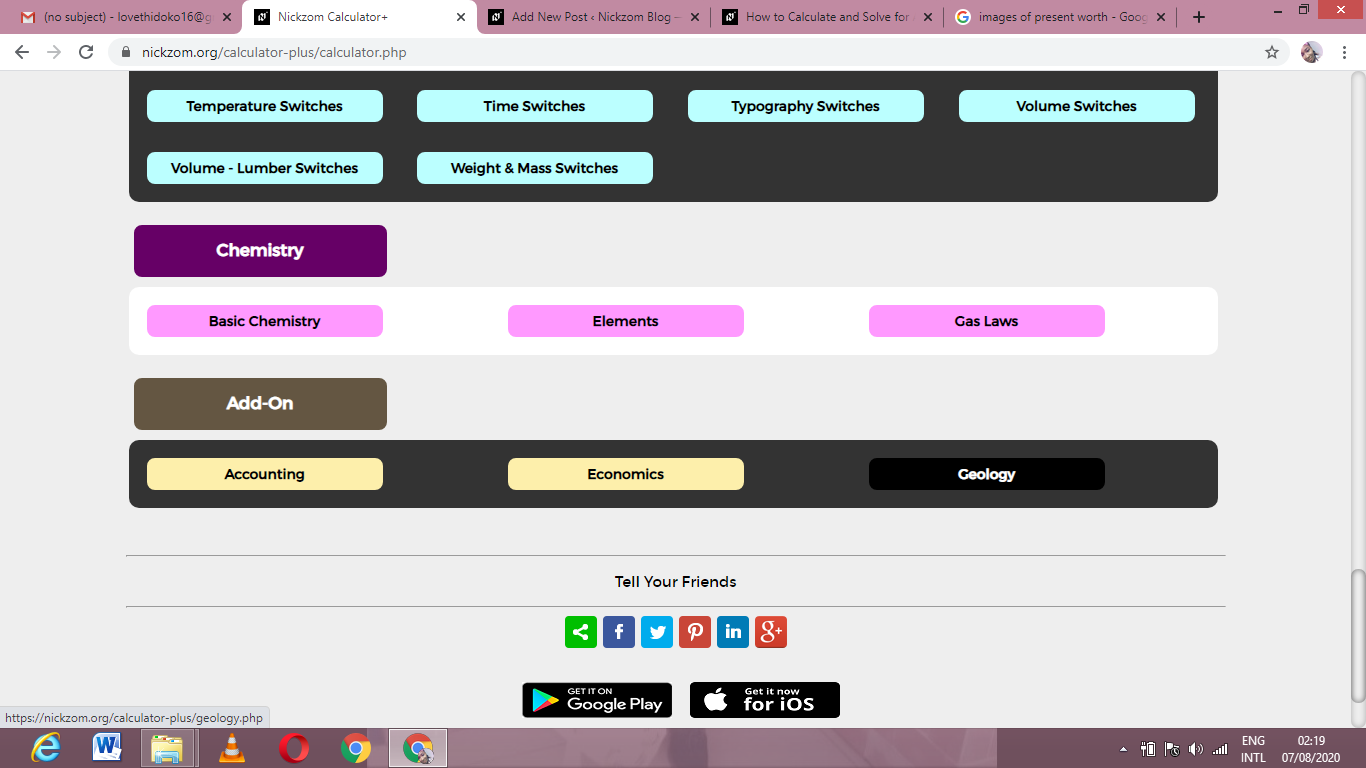Now, Click on Aquifer Characteristics under GeologyNow, Click on Pressure Head under Aquifer CharacteristicsThe screenshot below displays the page or activity to enter your values, to get the answer for the pressure head according to the respective parameters which are the Pressure (P), Density (ρ) and Acceleration due to Gravity (g).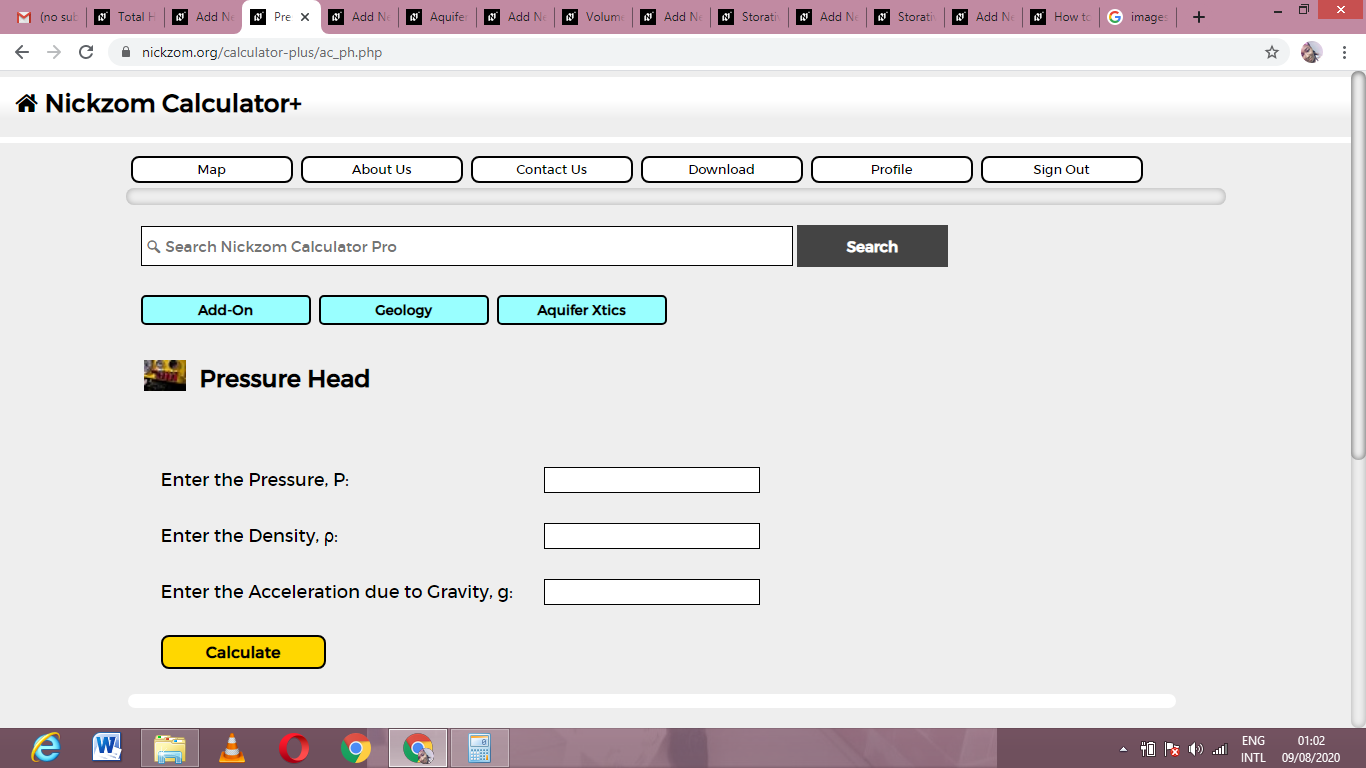Now, enter the values appropriately and accordingly for the parameters as required by the Pressure (P), Density (ρ) and Acceleration due to Gravity (g).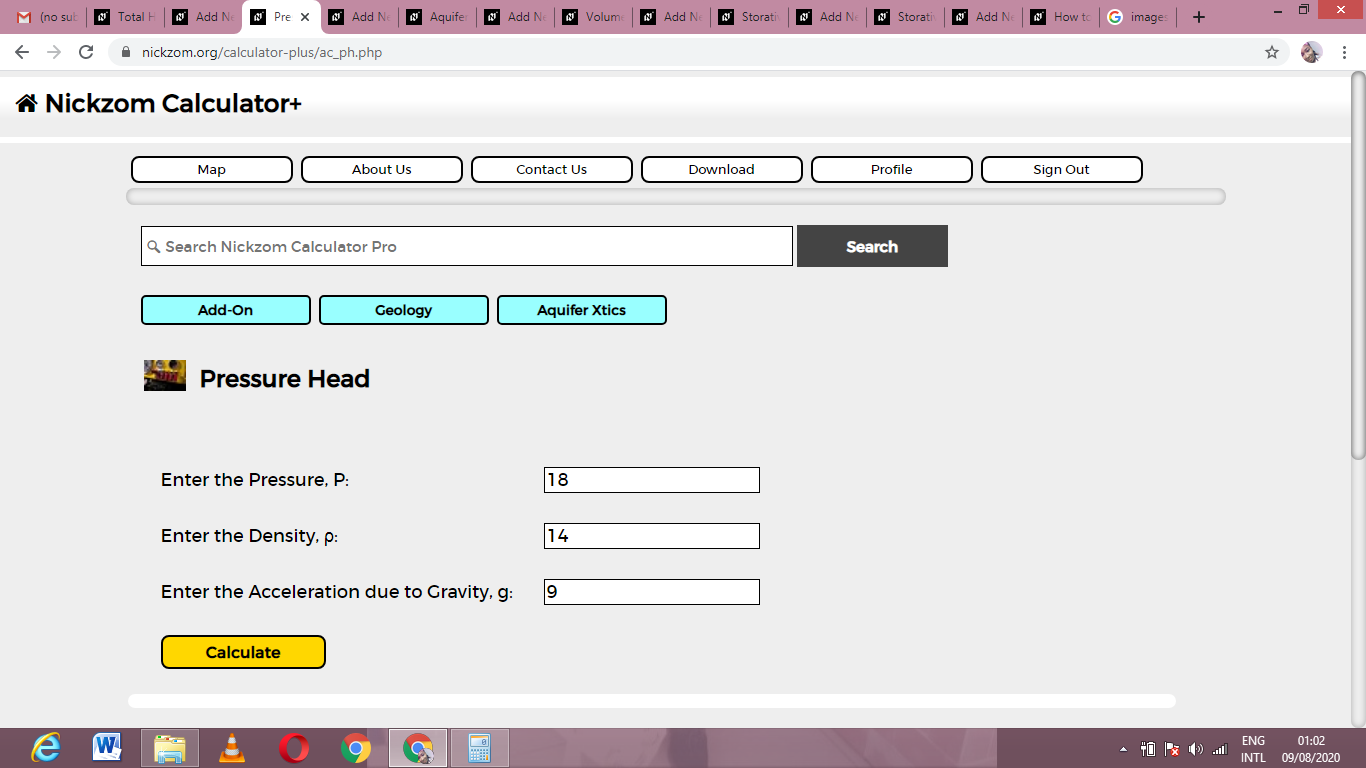Finally, Click on Calculate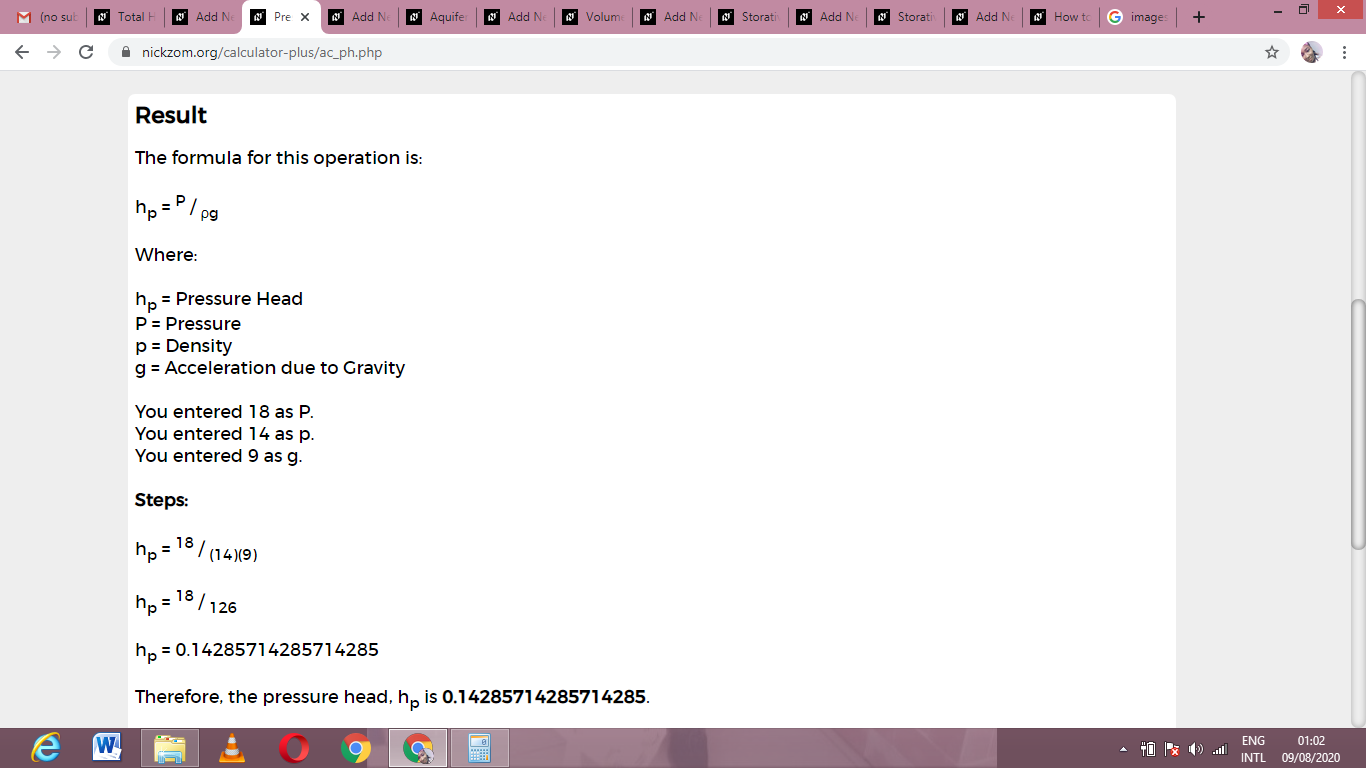As you can see from the screenshot above, Nickzom Calculator– The Calculator Encyclopedia solves for the pressure head and presents the formula, workings and steps too.